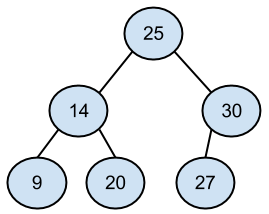# Mutable Trees: basic-level questions

If you need help reviewing Mutable Trees, take a look at these resources:

We will be using the OOP implementation of `Tree`s from lecture, found here

Each question has a "Toggle Solution" button -- click it to reveal that question's solution.

## Conceptual Questions

### Question 1

Define each of the following terms:

• entry/datum,
• node,
• child,
• parent,
• leaf,
• forest,
• binary search tree
• entry/datum: an item contained inside of a node
• node: a single "circle" or vertex in a tree that contains an item (the node is the container, the datum is the item inside that container).
• child: a node that has a parent (i.e. a node that branches off from another node)
• parent: a node that has at least one child (i.e. a node that has other nodes branching off from it
• leaf: a node that has no children (has no other nodes branching off underneath it).
• forest: one or more trees
• binary search tree: a type of tree that satisfies the following conditions:

1. each node can have at most 2 children, called a left and a right
2. every element in the subtree to the left of the node must be smaller than the element in the node.

### Question 2

Which of the following are valid `Tree` constructors?

1. Tree()
2. Tree(3)
3. Tree(5, [Tree(1), Tree(5)])
4. Tree(4, [Tree(2)])
5. Tree(2, [Tree(2, [4, 5])])
1. Invalid: trees must contain at least one element
2. Valid: this constructs a leaf with 3 as its entry
3. Valid: this constructs a tree whose datum is 5, and has two children whose elements are 1 and 5
4. Valid: this is a Tree that has one child
5. Invalid: the children of a tree must also be trees

### Question 3

Draw a graphical representation of the following tree and answer these three questions:

``````Tree(25,
[Tree(14,
[Tree(9),
Tree(20)]),
Tree(30,
[Tree(27)])])``````
1. What number is contained in the root of this tree?
2. Which numbers are contained in leaves?
3. How many children does the node containing 14 have?1. 25
2. 9, 20, 27
3. 2 children